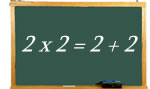Logic puzzles, riddles, math puzzles and brainteasers - pzzls.com

Vandaag is het 10 August 2022

# 2 x 2 = 2 + 2 - math puzzle

Difficulty:Rating: 2.9/5.0

 Two times two is equal to two and two. But are there more number pairs for which the sum of the numbers is is equal to the product of the numbers? The numbers may be different.# Explanation

Yes, there are even an infinite number of these pairs.

Let the numbers be x and y. So it must hold that
x * y = x + y
One can write this equation as
x = x/y + 1
From which easily follows that
y = x / (x - 1).

So as long as x is not equal to 1 one can find for any number x a number y such that x*y = x + y.
Take for example x = 5. Then y = 5/(5-1) = 1.25.
5 * 1.25 = 6.25
5 + 1.25 = 6.25